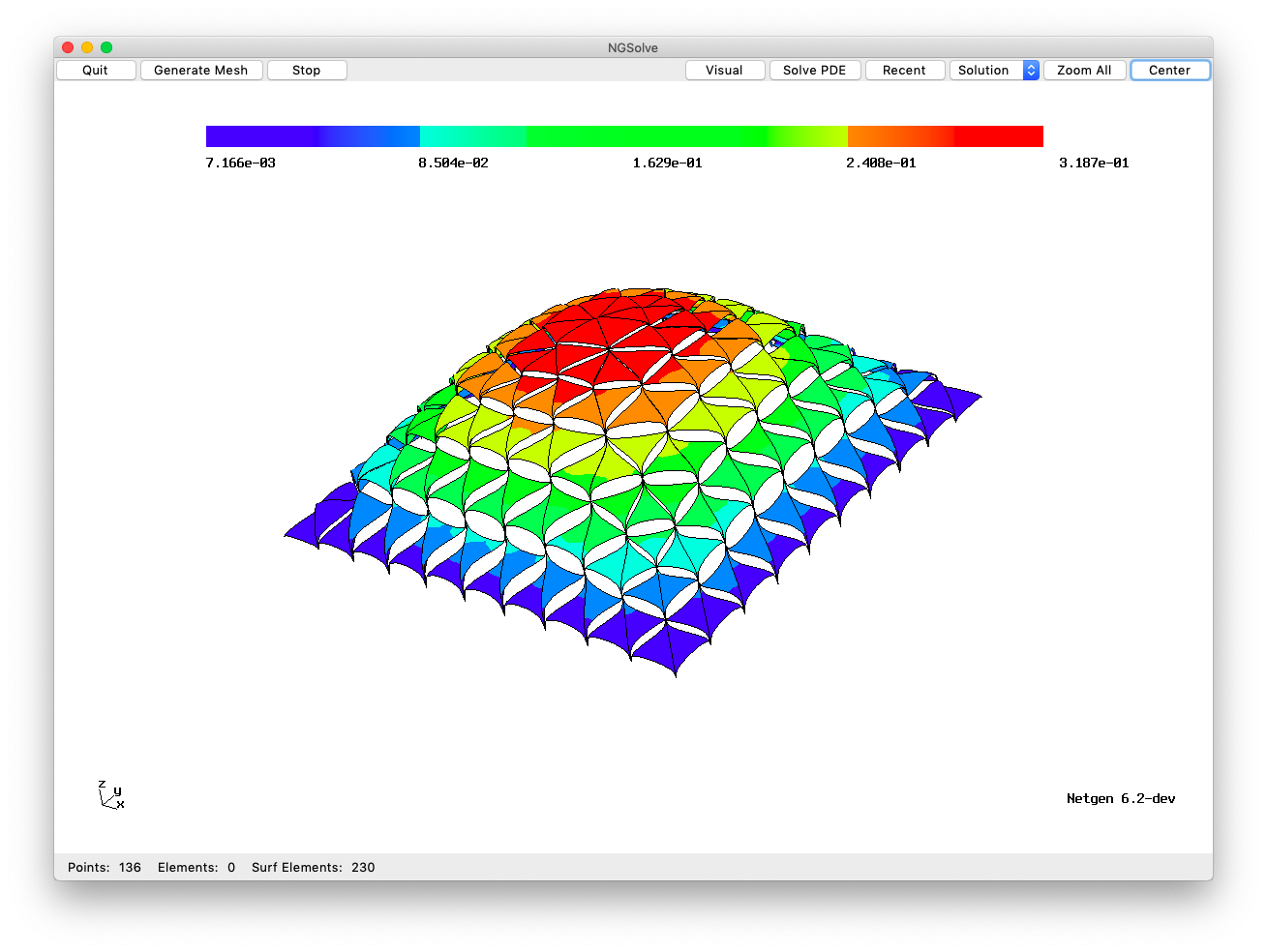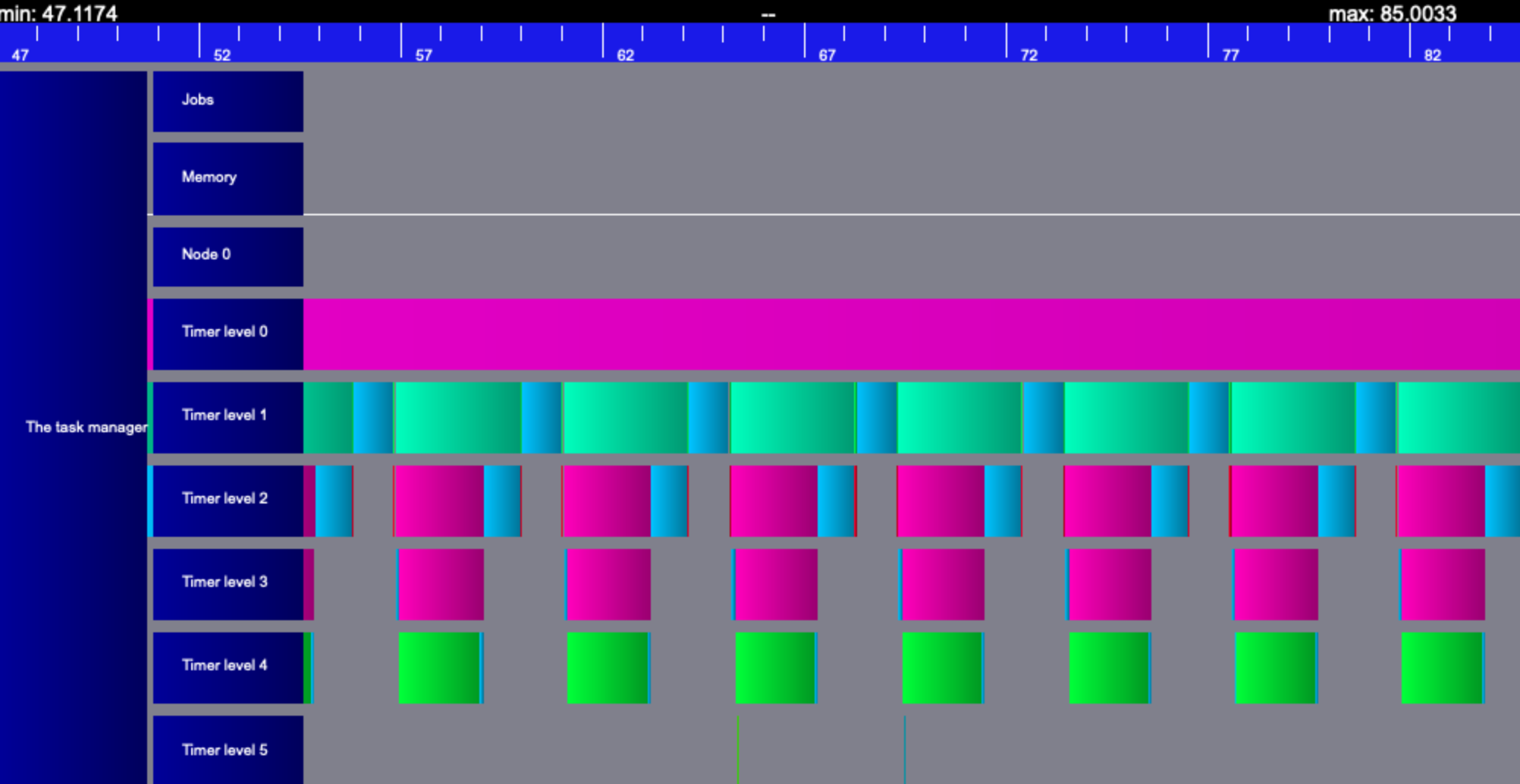# 5.5.1 Solving the Poisson Equation on devices¶

• After finite element discretization we obtain a (linear) system of equations.

• The new ngscuda module moves the linear operators to the Cuda - decice.

• The host is stearing, data stays on the device

• The module is now included in NGSolve Linux - distributions, and can be used whenever an accelerator card by NVIDIA is available, and the cuda-runtime is installed.

:

from ngsolve import *
from ngsolve.webgui import Draw
from time import time

:

mesh = Mesh(unit_square.GenerateMesh(maxh=0.1))
for l in range(5): mesh.Refine()
fes = H1(mesh, order=2, dirichlet=".*")
print ("ndof =", fes.ndof)

u, v = fes.TnT()
f = LinearForm(x*v*dx).Assemble()

gfu = GridFunction(fes)

jac = a.mat.CreateSmoother(fes.FreeDofs())

inv_host = CGSolver(a.mat, jac, maxiter=2000)
ts = time()
gfu.vec.data = inv_host * f.vec
te = time()
print ("steps =", inv_host.GetSteps(), ", time =", te-ts)

# Draw (gfu);

ndof = 476417
steps = 1751 , time = 9.355138301849365


Now we import the NGSolve - cuda library.

It provides

• an UnifiedVector, which allocates memory on both, host and device. The data is updated on demand either on host, or on device.

• NGSolve - matrices can create their counterparts on the device. In the following, the conjugate gradients iteration runs on the host, but all operations involving big data are performed on the accelerator.

:

try:
from ngsolve.ngscuda import *
except:
print ("no CUDA library or device available, using replacement types on host")

ngsglobals.msg_level=1
fdev = f.vec.CreateDeviceVector(copy=True)

no CUDA library or device available, using replacement types on host
No device creator function, creating host vector

:

adev = a.mat.CreateDeviceMatrix()
jacdev = jac.CreateDeviceMatrix()

inv = CGSolver(adev, jacdev, maxsteps=2000, printrates=False)

ts = time()
res = (inv * fdev).Evaluate()
te = time()

print ("Time on device:", te-ts)
diff = Norm(gfu.vec - res)
print ("diff = ", diff)

Time on device: 30.47527527809143
diff =  0.0


On an A-100 device I got (for 5 levels of refinement, ndof=476417):

Time on device: 0.4084775447845459 diff = 3.406979028373306e-12

## CG Solver with Block-Jacobi and exact low-order solver:¶

$\begin{split}A = \left( \begin{array}{cc} A_{cc} & A_{cf} \\ A_{fc} & A_{ff} \end{array} \right)\end{split}$

$C^{-1} = P A_{cc}^{-1} P^T + \sum_i E_i A_i^{-1} E_i^T$

with * $$P$$ .. embedding of low-order space * $$A_i$$ .. blocks on edges/faces/cells * $$E_i$$ .. embedding matrices

:

fes = H1(mesh, order=5, dirichlet=".*")
print ("ndof =", fes.ndof)

u, v = fes.TnT()
f = LinearForm(x*v*dx).Assemble()

gfu = GridFunction(fes)

jac = a.mat.CreateBlockSmoother(fes.CreateSmoothingBlocks())
lospace = fes.lospace
loinv = a.loform.mat.Inverse(inverse="sparsecholesky", freedofs=lospace.FreeDofs())
loemb = fes.loembedding

pre = jac + loemb@loinv@loemb.T
print ("mat", a.mat.GetOperatorInfo())
print ("preconditioner:")
print(pre.GetOperatorInfo())

inv = CGSolver(a.mat, pre, maxsteps=2000, printrates=False)
ts = time()
gfu.vec.data = inv * f.vec
te = time()
print ("iterations =", inv.GetSteps(), "time =", te-ts)

ndof = 2972801
mat SparseMatrixd, h = 2972801, w = 2972801

preconditioner:
SumMatrix, h = 2972801, w = 2972801
BlockJacobi-d, h = 2972801, w = 2972801
EmbeddedTransposeMatrix, h = 2972801, w = 2972801
EmbeddedMatrix, h = 2972801, w = 119425
SparseCholesky-d, h = 119425, w = 119425

iterations = 37 time = 5.43648624420166

:

adev = a.mat.CreateDeviceMatrix()
predev = pre.CreateDeviceMatrix()
fdev = f.vec.CreateDeviceVector()

inv = CGSolver(adev, predev, maxsteps=2000, printrates=False)
ts = time()
gfu.vec.data = (inv * fdev).Evaluate()
te = time()
print ("iterations =", inv.GetSteps(), "time =", te-ts)

No device creator function, creating host vector
iterations = 37 time = 5.384337663650513


on the A-100:

SumMatrix, h = 2896001, w = 2896001 N4ngla20DevBlockJacobiMatrixE, h = 2896001, w = 2896001 EmbeddedTransposeMatrix, h = 2896001, w = 2896001 EmbeddedMatrix, h = 2896001, w = 116353 N4ngla17DevSparseCholeskyE, h = 116353, w = 116353 iterations = 37 time= 0.6766986846923828

## Using the BDDC preconditioner:¶

For the BDDC (balancing domain decomposition with constraints) preconditioning, we build a FEM system with relaxed connectivity:This allows for static condensation of all local and interface degrees of freedom, only the wirebasket dofs enter the global solver. The resulting matrix $$\tilde A$$ is much cheaper to invert.

The preconditioner is

$P = R {\tilde A}^{-1} R^T$

with an averagingn operator $$R$$.

:

mesh = Mesh(unit_square.GenerateMesh(maxh=0.1))
for l in range(3): mesh.Refine()
fes = H1(mesh, order=10, dirichlet=".*")
print ("ndof =", fes.ndof)

u, v = fes.TnT()
pre = Preconditioner(a, "bddc")
a.Assemble()
f = LinearForm(x*v*dx).Assemble()

gfu = GridFunction(fes)
inv = CGSolver(a.mat, pre, maxsteps=2000, printrates=False)
ts = time()
gfu.vec.data = (inv * f.vec).Evaluate()
te = time()
print ("iterations =", inv.GetSteps(), "time =", te-ts)

 Mesh bisection
Bisection done
Mesh bisection
Bisection done
Mesh bisection
Bisection done
ndof = 744001
iterations = 53 time = 3.4315502643585205

:

predev = pre.mat.CreateDeviceMatrix()
print (predev.GetOperatorInfo())

SumMatrix, h = 744001, w = 744001
ProductMatrix, h = 744001, w = 744001
ProductMatrix, h = 744001, w = 744001
SparseMatrixd, h = 744001, w = 744001
SparseCholesky-d, h = 744001, w = 744001
SparseMatrixd, h = 744001, w = 744001
SparseMatrixd, h = 744001, w = 744001


:

adev = a.mat.CreateDeviceMatrix()
predev = pre.mat.CreateDeviceMatrix()
fdev = f.vec.CreateDeviceVector()

inv = CGSolver(adev, predev, maxsteps=2000, printrates=False)
gfu.vec.data = (inv * fdev).Evaluate()
print ("iterations =", inv.GetSteps())

No device creator function, creating host vector
iterations = 52

A100: iterations = 53 time= 0.16417622566223145

Vite - traces:[ ]: